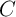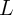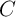### 6.1Overview

The memristor concept has attracted strong attention due to its practical demonstration based on different technologies like metal/oxide/metal thin-film and spintronic. It has the potential to lead to novel applications beyond non-volatile memory due to its functional properties, which are not accessible in electronic circuits combining resistors, capacitors, and inductors. As the fourth fundamental circuit element, the memristor is potentially suited for a wide range of tasks (Chapter 1). In this Chapter, it is shown how the unique ability of the memristor to memorize the history of the applied current or voltage leads to novel sensing capabilities which cannot be achieved by RLC-networks alone. The historic profile of the applied current or voltage memorized in the memristance change can be revealed instantaneously by keeping track of its varying resistance. In principle, this storage capability, which is independent of the memristor material, can reduce the charge (flux)-based capacitance (inductance) sensing to a simple resistance measurement.

The behavior of the basic electrical circuits are determined by Kirchhoff’s current (KCL) and voltage (KVL) laws. A resistor relates the voltage and the current by a (linear) relationship called Ohm’s law. Its resistance, therefore, can be determined by measuring the current and the voltage simultaneously. Since a capacitor and an inductor relate their voltage and current through differential equations (Section 2.1.1), the capacitance and the inductance are typically measured indirectly and their measurement techniques are entirely different from those used for a resistor. Approaches to measuringandare based on time domain techniques and frequency domain techniques. For example, in the time domain techniquesis calculated by measuring the oscillation period of a relaxation oscillator [204, 205] or the time required to reach a threshold voltage in a charge-discharge circuit [206, 207]. In the frequency domain technique an AC signal of a known frequency is applied to a capacitive divider (CC circuit) or an RC circuit. The magnitude and the phase of the output signal across the capacitor or the resistor are measured to determine[208, 209].